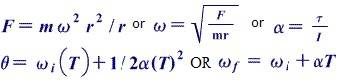# Pendulum on a tower

• ataglance05

## Homework Statement

A 50 meter tower is on a flat plane. A 10 kg motor is tethered to the top of the tower by a 100 meter wire. The motor fires with sufficient force that has a constant angular velocity of .75 rad/sec.

1) What is the tension in Newtons on the tether? I think one uses F=mω2r2/r
2) How high off the ground is the object as it spins around the tower?
3) How does the angle that the tether makes with the horizontal vary with the angular velocity? (In other words: What is the equation that relates the angle to the angular velocity?)

## Homework Equations## The Attempt at a Solution

Not sure if any of these are right:

1) F=m^2r^2/r
=10(.75)(100)
=750 N
2) Highest point off the ground is 50m because that's how high the tower is.
3)The angle is directly proportional to the angular velocity. (The equation is theta=ωi(T)+1/2(alpha)(T)^2

You mean that a 10kg object is tethered to a motor atop a tower, right? You need to draw a force (and geometry) diagram and split into components. Both the tension AND GRAVITY are acting on the circling object. That's why the angle of the tether isn't horizontal.